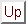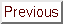Next: Remarks about the constrained Up: Detailed description of the Previous: The SQP algorithm   Contents

# The constrained step in detail

The stepof the constrained algorithm are the solution of: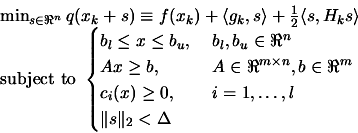We will use a null-space, active set approach. We will follow the notations of section 9.1.1.
1. Let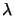be a vector of Lagrange Multiplier associated with all the linear constraints. This vector is recovered from the previous calculation of the constrained step. Set,The constraints which are active are determined by a non-null,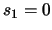. If aassociated with a non-linear constraint is not null, set NLActive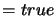, otherwise set NLActive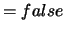.
2. Compute the matrixandassociated with the reduced space of the active box and linear constraints. The active set is determined by.
3. We will now compute the step in the reduced-space of the active box and linear constraints. Check NLActive:
• True: We will use the SQP algorithm described in the previous section to compute the step. Once the step is calculated, check the Lagrange Multipliers associated with the non-linear constraints. If they are all null or negative, set NLActive.
• False: We will use the Dennis-Moré algorithm of chapter 4 to compute the step. The stepis computed using: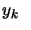is the solution of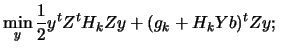subject to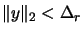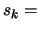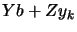Where the trust region radiusused in the reduced spaced isas illustrated in figure 9.6.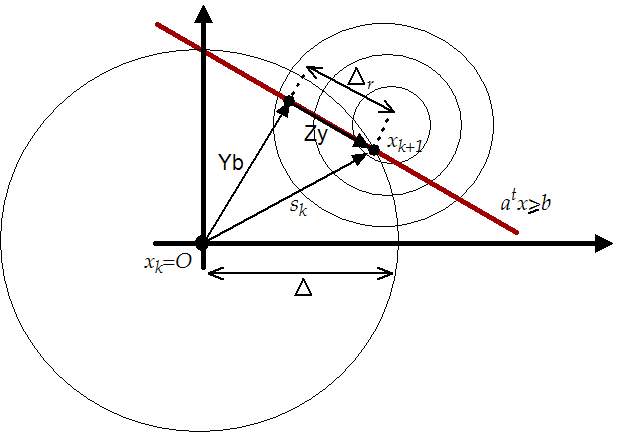4. Compute the Lagrange multipliers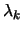. Iffor all constraints then terminate. Remove fromthe constraints which have negative.
5. Check if a non-linear constraint has been violated. If the test is true, set NLActive, set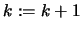and go to (2).
6. Solve 9.13 and add if necessary a new box or linear constraint inside. Setand go to (2).
This is really a small, simple sketch of the implemented algorithm. The real algorithm has some primitive techniques to avoid cycling. As you can see, the algorithm is also able to "warm start", using the previouscomputed at the previous step.Next: Remarks about the constrained Up: Detailed description of the Previous: The SQP algorithm   Contents
Frank Vanden Berghen 2004-04-19